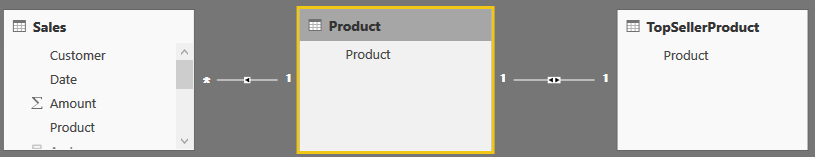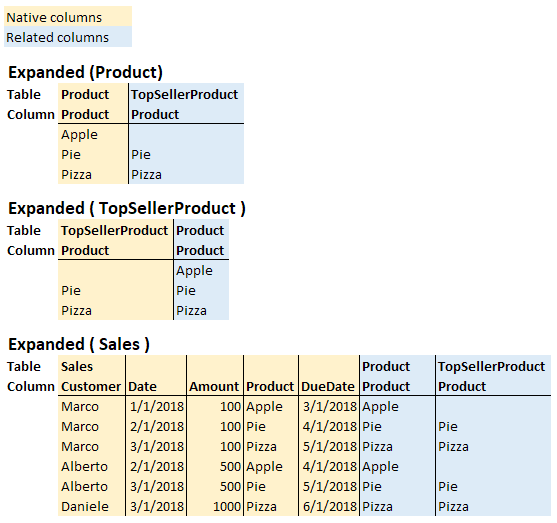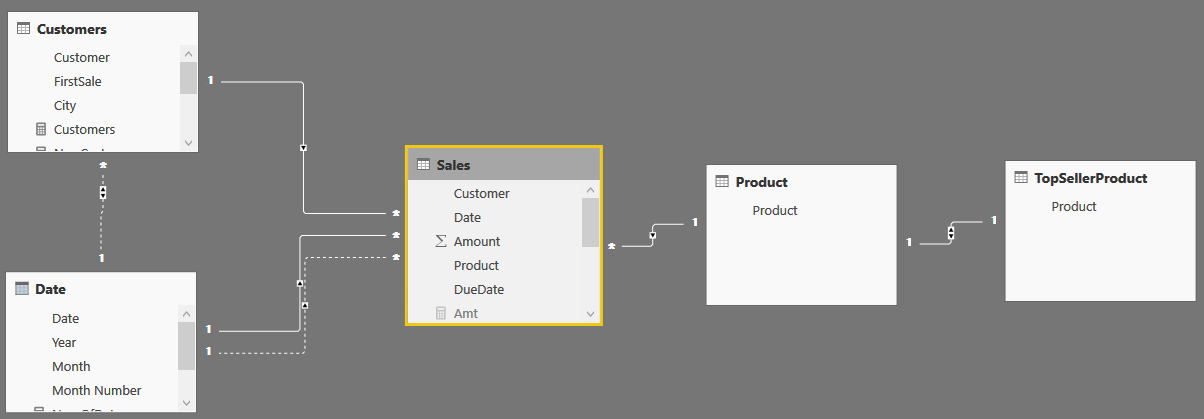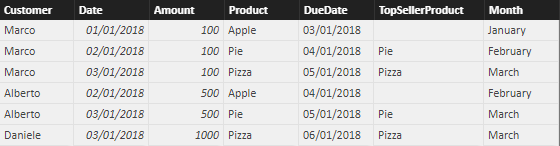An expanded table contains all the columns of the base table and all the columns of the tables that are linked to the base table through one or more cascading many-to-one or one-to-one relationships.

Consider the following diagram:There are three tables. Each one has its expanded version:• Expanded ( Product ) contains Product[Product] and TopSellerProduct[Product]
• Expanded ( TopSellerProduct ) contains Product[Product] and TopSellerProduct[Product]
• Expanded ( Sales ) contains all the columns of the three tables

The expanded version of both Product and TopSellerProduct is the same. In fact, a one-to-one relationship is known as an identity. For all intents and purposes you can consider the two expanded tables as being the same. An expanded table is created by joining the columns of two tables into a larger table using a FULL OUTER JOIN. However, regular many-to-one relationships use the usual LEFT OUTER JOIN.

Table expansion has nothing to do with bidirectional filtering. Expansion always happens to the 1-side of a relationship. If you activate the bidirectional cross-filter on a relationship, you are not relying on table expansion. Instead, the engine pushes certain filtering conditions in the code in order to apply the filters on both sides. Thus, in the previous model, if you enable bidirectional cross-filter on the relationship between Sales and Product, this will not add the columns of the Sales table to the expanded Product table.

Each expanded table contains both native and related columns. Native columns are the ones originally present in the table. Related columns are all the columns of related tables, added to the original table through table expansion.

Table expansion does not happen physically. The VertiPaq engine only stores native tables. Nevertheless, the whole DAX semantic is based on the theoretical concept of expanded tables.

## Filter propagation

When you learn the CALCULATE function, you learn that applying a filter on the one-side of a relationship affects the many-side. In fact, if you write this measure:

```AppleSales :=
CALCULATE (
SUM ( Sales[Amount] ),
Product[Product] = "Apple"
)
```

The filter applied on Product[Product] follows the relationship between Product and Sales, thus filtering the Sales table too. A better description of that same filter propagation uses the concept of expanded tables. When you filter Product[Product], all the tables that contain that column – either native or related – are filtered.
Thus, Sales is filtered by Product[Product] because the expanded version of Sales contains Product[Product].

## RELATED, RELATEDTABLE and table expansion

Table expansion includes the concept of relationship. In fact, a relationship is used when the table is expanded and, once you start thinking in terms of expanded tables, you no longer need to think about relationships.

Consider the RELATED function. When beginning to learn DAX, one typically thinks that RELATED lets you access columns in related tables. A more accurate way of looking at this is that RELATED lets you access the related columns of an expanded table.

As an example, consider the following model:There are direct relationships between Sales, and Product, Date, and Customer. In more appropriate DAX language, we would say that the expanded version of Sales includes all columns of Product, Date and Customer. Thus, Product[Product] belongs to the expanded version of Sales. The expanded version of Sales includes the entire model. Therefore, you could author two columns in Sales using the RELATED function, like this:

```Sales[TopSellerProduct] = RELATED ( TopSellerProduct[Product] )
Sales[Month] = RELATED ( 'Date'[Month] )
```

The result is the following:Not all the products are top sellers, which is why there is a blank value for sales of Apple products in the TopSellerProduct column.

If you are coming from an SQL background, or if you are used to relational databases, you probably think that RELATED follows relationships. Thus, to compute the Month column, you would think that the engine followed a relationship between Sales and Date and obtained the value of the month by performing a lookup on the Date table.

DAX is different. Date[Month] belongs to the expanded version of Sales, There is a value for RELATED(Date[Month]) because Sales was expanded to include Date using a relationship.
RELATED requires a row context to be active. If you remove the row context of the calculated column, then RELATED no longer works. For example, the following calculated column raises an error because CALCULATE removes the row context performing a context transition:

```Sales[Wrong] = CALCULATE ( RELATED ( TopSellerProduct[Product] ) )
```

## Table expansion and variables

One important rule about table expansion is that it happens when you define a table. Look, for example, at the following query:

```DEFINE
VAR SalesA =
CALCULATETABLE ( Sales, USERELATIONSHIP ( Sales[Date], 'Date'[Date] ) )
VAR SalesB =
CALCULATETABLE ( Sales, USERELATIONSHIP ( Sales[DueDate], 'Date'[Date] ) )
EVALUATE
ADDCOLUMNS ( SalesB, "Month", RELATED ( 'Date'[Month] ) )
```

The two variables store the Sales table using two different relationships. SalesA uses the default relationship, whereas SalesB uses the relationship with Sales[DueDate] instead of Sales[Date]. The last ADDCOLUMNS iterates SalesB and returns the RELATED Date[Month]. What will the result be? The month of the Sales[Date] column or the month of the Sales[DueDate] column? If you are still thinking in terms of relationships, you are in trouble. In fact, when ADDCOLUMNS is executed, the active relationship is the relationship using Sales[Date] and you would think that the month is the month of that date. Right? Wrong!

The correct reasoning is as follows: RELATED accesses the related columns of the expanded version of Sales. SalesB contains the expanded Sales table, and that expansion happened when the active relationship was the relationship with Sales[DueDate]. As a result, Date[Month] contained in SalesB is related to Sales[DueDate], not to Sales[Date]. Obviously, if you iterate over SalesA, the result will be different.

## RELATED in calculated columns

If the developer needs to obtain a RELATED column using an inactive relationship in a calculated column, they would be in trouble. In fact, as we demonstrated, one could activate an inactive relationship using USERELATIONSHIP. However, this requires using CALCULATE which in turn, destroys the row context. Thus, the following definition of a calculated column would generate an error:

```Sales[DueMonth] =
CALCULATE (
RELATED ( 'Date'[Month] ),
USERELATIONSHIP ( 'Date'[Date], Sales[DueDate] )
)
```

The error is introduced by CALCULATE, which inhibits the usage of RELATED. It would be great if specifying USERELATIONSHIP as part of RELATED was an option, but as of today this syntax is unavailable in DAX. RELATED always uses the active relationship and there is no way to specify an alternative relationship as part of its syntax.

A possible solution is to introduce a row context after USERELATIONSHIP changes the active relationship – so that table expansion happens with a different set of active relationships. This version of Sales[DueMonth] provides the correct result, although it is not very efficient:

```Sales[DueMonth] =
CALCULATE (
MINX ( Sales, RELATED ( 'Date'[Month] ) ),
USERELATIONSHIP ( Sales[DueDate], 'Date'[Date] ),
ALL ( 'Date' )
)
```

This code is very intricate, and we urge motivated readers to follow the details of how it works: CALCULATE activates the new relationship through USERELATIONSHIP; ALL on Date is required in order to get rid of the filter moved to Date by the context transition executed by CALCULATE. In fact, the context transition still operates using the original relationship and its effect needs to be removed. The inner MINX reintroduces a row context, in order to use RELATED to retrieve the date from the Sales expanded table. Obviously, the expansion happened when the active relationship was the one needed. Looks intricate, right? It is… In fact, the suggestion is to never write code like this. It is present in the article for educational purposes but, if one ever needs a piece of code like this, it is much better to rely on a simpler version, which does not use relationships at all:

```Sales[DueMonth] =
LOOKUPVALUE (
'Date'[Month],
'Date'[Date],
Sales[DueDate]
)
```

This latter version is faster and safer. Yet, if one wants to master relationships, it is important to also understand the previous version. Understand it – but never use it, of course.

Before leaving the topic, it is worth discussing why this alternative version of the code for the due month does not work:

```Sales[DueMonth] =
CALCULATE (
VALUES ( 'Date'[Month] ),
USERELATIONSHIP ( 'Date'[Date], Sales[DueDate] ),
ALL ( 'Date' )
)
```

It looks very similar to the code #6, which works. This time however, it is using VALUES instead of a less elegant MINX. The reason why this code is not working is that USERELATIONSHIP only changes the active relationship in the model, so that the table expansion inside of CALCULATE uses the newly activated relationship instead of the default relationship. USERELATIONSHIP, by itself, does not introduce a filter. It only activates a relationship.

Thus, context transition happens with the old relationship in place. ALL(‘Date’) removes its effect on the Date table, but USERELATIONSHIP does not transfer the filter to the Date table. As a result, all dates are still visible. For example, if you were to apply a filter manually – by using TREATAS instead of USERELATIONSHIP – then the code would work fine although it would not rely on table expansion:

```Sales[DueMonth] =
CALCULATE (
VALUES ( 'Date'[Month] ),
TREATAS ( { Sales[DueDate] }, 'Date'[Date] )
)
```

In this case, TREATAS introduces a filter by using the current value of DueDate, and it moves it as a new filter to Date[Date]. Lastly, it is worth to note that – in this case – ALL on the Date table is no longer needed, because the new filter introduced by TREATAS overrides it.

## Conclusions

Table expansion is a unique concept introduced in DAX, which incorporates the notion of relationships. Though it seems strange in the beginning, it becomes very natural once you get used to it. We have written several articles that reference table expansion as the vital concept required to understand why DAX behaves a certain way. You can look, for example, at Managing all functions in dax, Filtering tables and Context transition and expanded tables. Still, an article describing what expanded tables are was missing on our website.
The purpose of this article is not that of solving a specific issue. Instead, we wanted to urge our readers to start (or continue) thinking in terms of expanded tables whenever they look at code. DAX offers many advanced features that we will be able to explore further once the concept of expanded tables is well understood.

LEFTReturns the specified number of characters from the start of a text string.

`LEFT ( <Text> [, <NumberOfCharacters>] )`

CALCULATEContext transition

Evaluates an expression in a context modified by filters.

`CALCULATE ( <Expression> [, <Filter> [, <Filter> [, … ] ] ] )`

RELATEDTABLEContext transition

Returns the related tables filtered so that it only includes the related rows.

`RELATEDTABLE ( <Table> )`

ADDCOLUMNSReturns a table with new columns specified by the DAX expressions.

`ADDCOLUMNS ( <Table>, <Name>, <Expression> [, <Name>, <Expression> [, … ] ] )`

USERELATIONSHIPCALCULATE modifier

Specifies an existing relationship to be used in the evaluation of a DAX expression. The relationship is defined by naming, as arguments, the two columns that serve as endpoints.

`USERELATIONSHIP ( <ColumnName1>, <ColumnName2> )`

ALLCALCULATE modifier

Returns all the rows in a table, or all the values in a column, ignoring any filters that might have been applied.

`ALL ( [<TableNameOrColumnName>] [, <ColumnName> [, <ColumnName> [, … ] ] ] )`

MINXReturns the smallest value that results from evaluating an expression for each row of a table. Strings are compared according to alphabetical order.

`MINX ( <Table>, <Expression> )`

VALUESWhen a column name is given, returns a single column table of unique values. When a table name is given, returns a table with the same columns and all the rows of the table (including duplicates) with the additional blank row if present.

`VALUES ( <TableNameOrColumnName> )`

TREATASTreats the columns of the input table as columns from other tables.For each column, filters out any values that are not present in its respective output column.

`TREATAS ( <Expression>, <ColumnName> [, <ColumnName> [, … ] ] )`